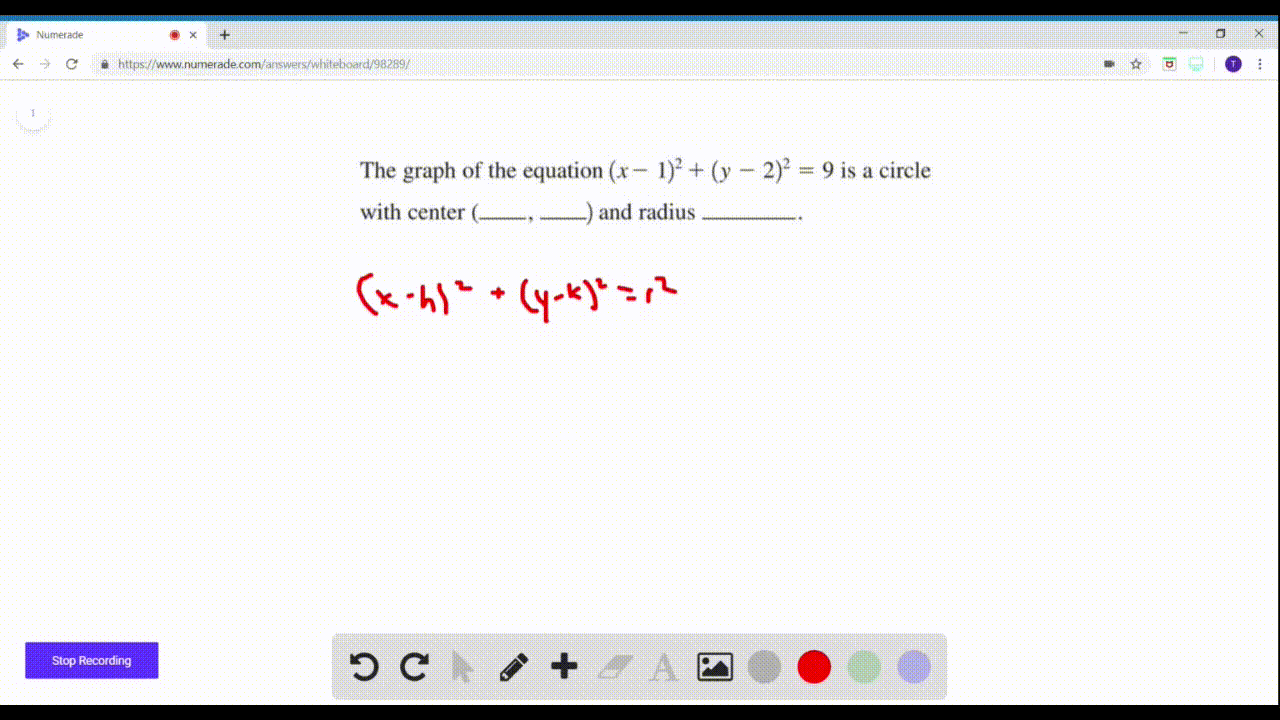🎉 The Study-to-Win Winning Ticket number has been announced! Go to your Tickets dashboard to see if you won! 🎉View Winning Ticket### The graph of the equation $(x-1)^{2}+(y-2)^{2}=9$…

01:18Problem 3

# To find the $y$ -intercept(s) of the graph of an equation, we set _____ equal to 0 and solve for _____. So the $y$ -intercept of $2 y=x+1$ is _____.

## Discussion

You must be signed in to discuss.

## Video Transcript

this item asks to find the Y intercept off the graph of an equation, we sit X equal to zero. And so, for why so Here, given the equation to y equals X plus one, we set X equal to zero. It's so for why and therefore the Y intercept noted as in order to pair is 0 1/2